Ex 7.2

Chapter 7 Class 10 Coordinate Geometry
Serial order wise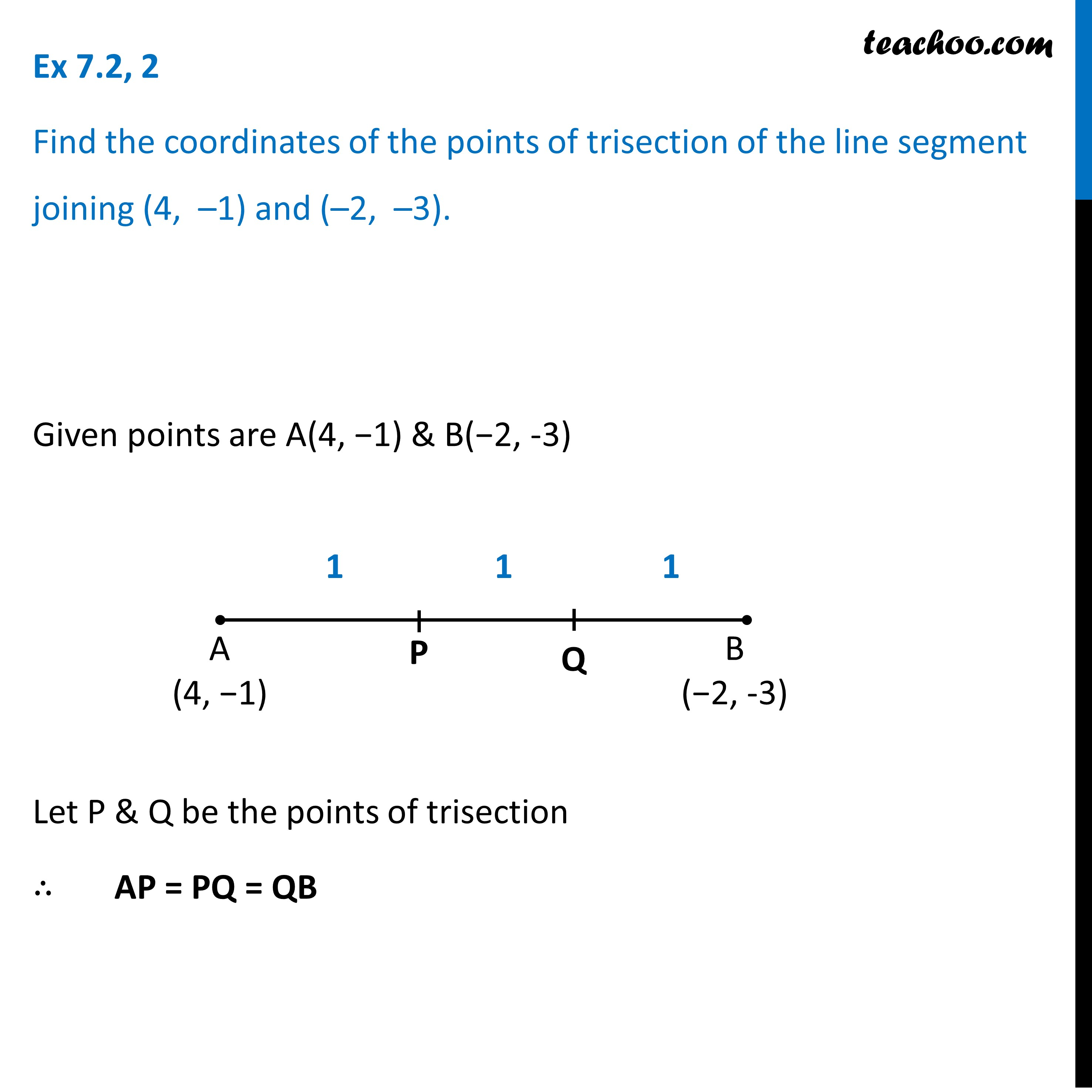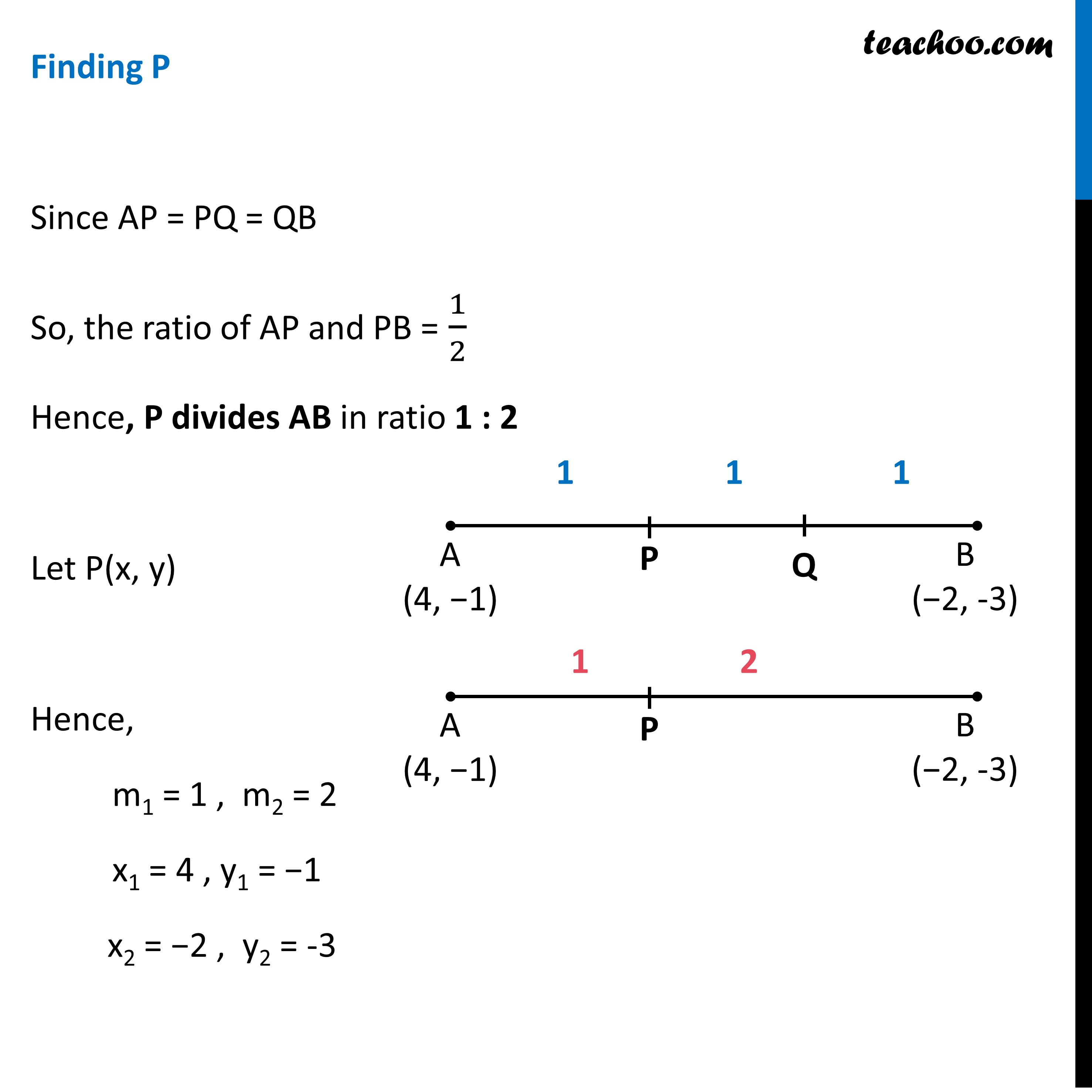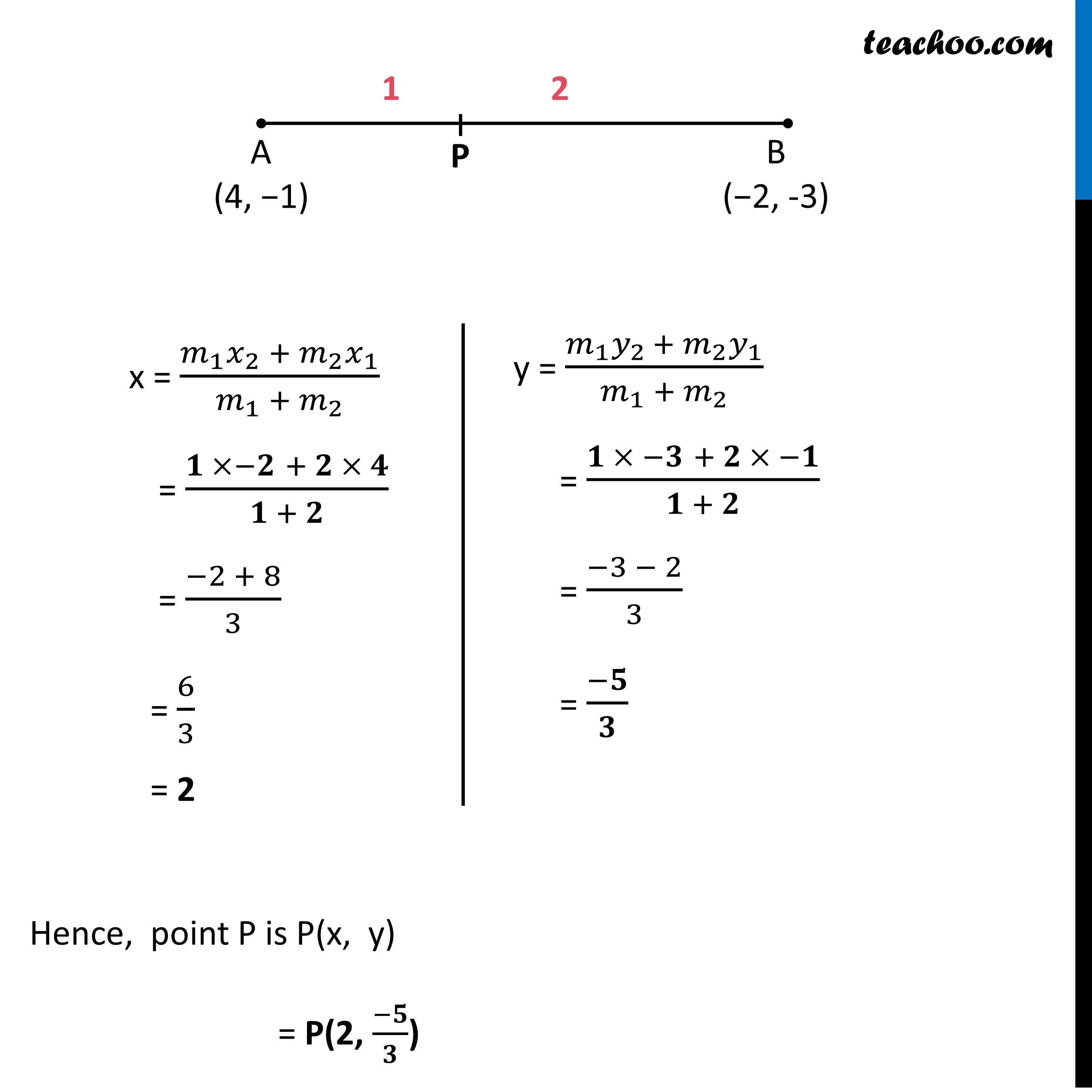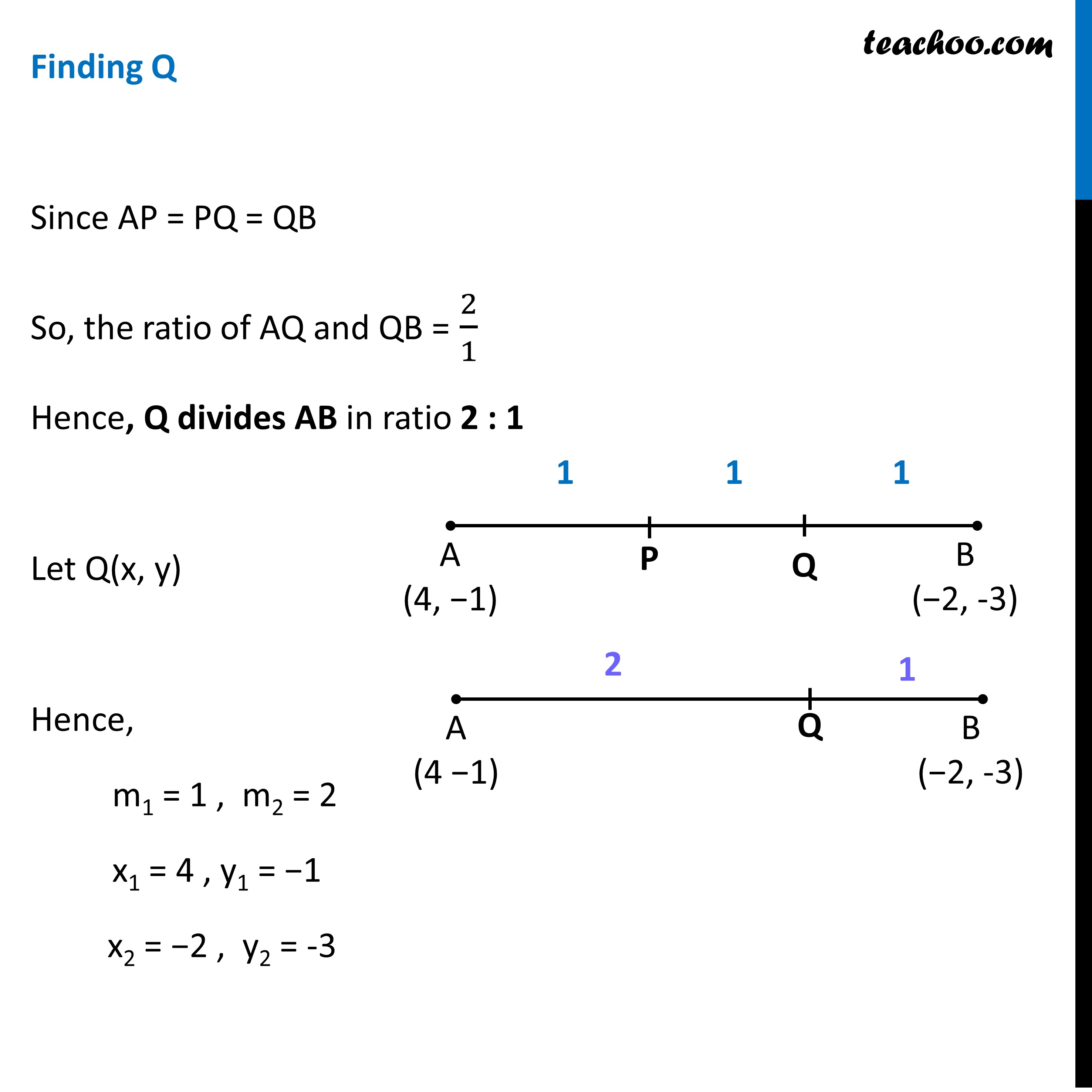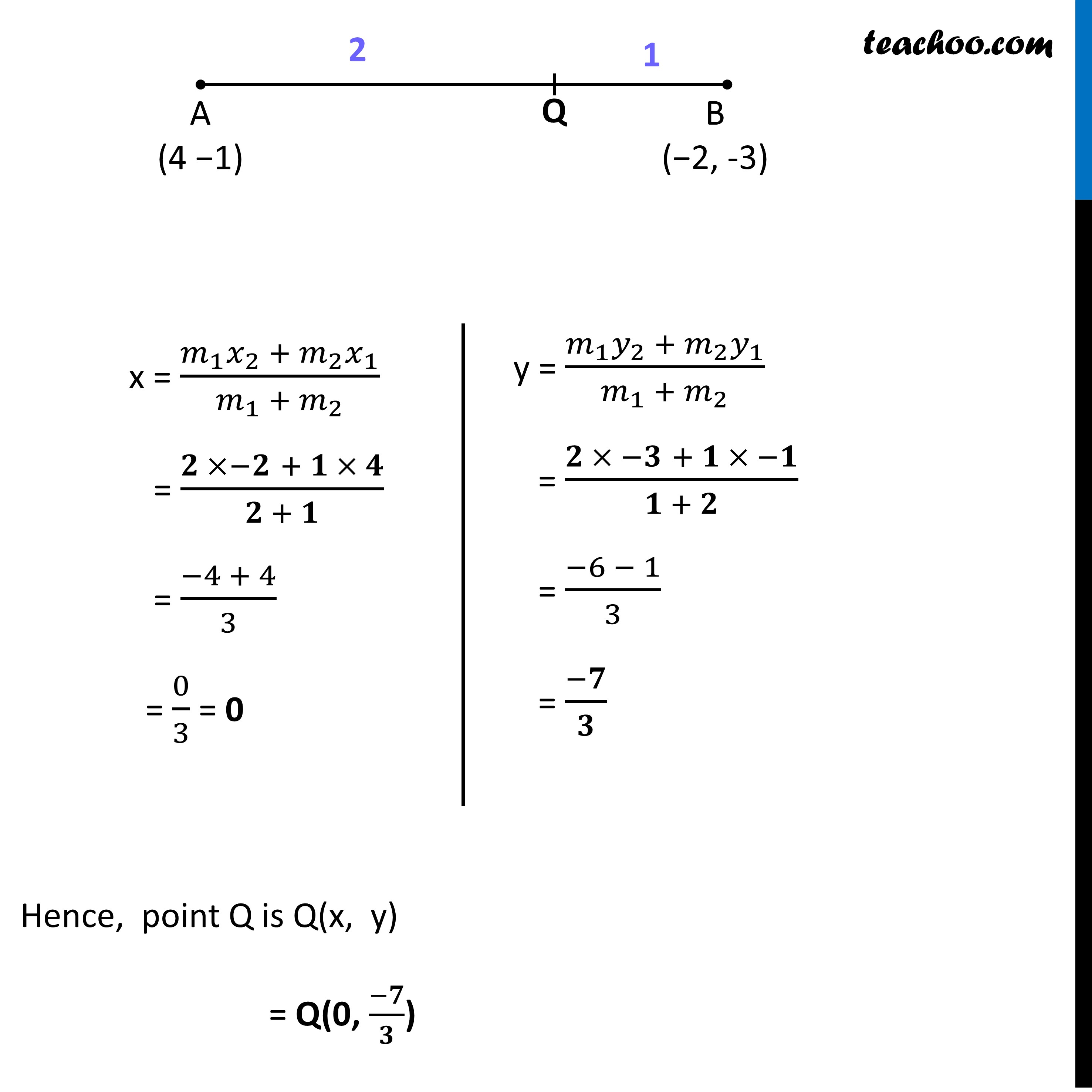Learn in your speed, with individual attention - Teachoo Maths 1-on-1 Class

### Transcript

Ex 7.2, 2 Find the coordinates of the points of trisection of the line segment joining (4, –1) and (–2, –3). Let the given points be A (4, −1) & B (−2, 3) P & Q are two points on AB such that AP = PQ = QB Let AP = PQ = QB = m Point P divides AP & PB in the ratio AP = m PB = PQ + QB = k + k = 2k Hence, Ratio between AP & PB = AP/PB = 𝑘/2𝑘 = 1/2 Thus P divides AB in the ratio 1:2 Finding P Let P(x, y) m1 = 1, m2 = 2 And for AB x1 = 4, x2 = −2 y1 = −1, y2 = −3 x = (𝑚_1 𝑥_2 + 𝑚_2 𝑥_1)/(𝑚_1 + 𝑚_2 ) = (1 ×−2 + 2 × 4)/(1 + 2) = (−2 + 8)/3 = 6/3 = 2 y = (𝑚_1 𝑦_(2 )+ 𝑚_2 𝑦_1)/(𝑚_1 + 𝑚_2 ) = (1 × −3 + 2 × −1)/(1 + 2) = (−3 − 2)/3 = (−5)/3 Hence, point P is P(x, y) = P ("2, " (−5)/3) Similarly, Point Q divides AB in the ratio AQ & QB 𝐴𝑄/𝑄𝐵 = (𝐴𝑃 + 𝑃𝑄)/𝑄𝐵 = (𝑘 + 𝑘 )/𝑘 = 2𝑘/𝑘 = 2/1 = 2 : 1 Finding Q Let Q be Q(x , y) m1 = 2, m2 = 1 x1 = 4, x2 = −2 y1 = −1, y2 = −3 x = (𝑚_1 𝑥_2 + 𝑚_2 𝑥_1)/(𝑚_1 + 𝑚_2 ) = (2 ×−2 + 1 × 4)/(2 + 1) = (−4 + 4)/3 = 0/3 = 0 y = (𝑚_1 𝑦_2 + 𝑚_2 𝑦_1)/(𝑚_1 + 𝑚_2 ) = (2 × −3 + 1 × −1)/(1 + 2) = (−6 − 1)/3 = (−7)/3 Hence, point Q is Q(x, y) = Q ("0, " (−𝟕)/𝟑)